# 1st PUC Physics Question Bank Chapter 4 Motion in a Plane

## Karnataka 1st PUC Physics Question Bank Chapter 4 Motion in a Plane

### 1st PUC Physics Motion in a Plane TextBook Questions and Answers

Question 1.
State, for each of the following physical quantities, if it is a scalar or a vector: volume, mass, speed, acceleration, density, number of moles, velocity, angular frequency, displacement, angular velocity.
Scalar: Volume, mass, speed, density, number of moles, angular frequency.
Vector: Acceleration, velocity, displacement, angular velocity.

Question 2.
Pick cut the two scalar quantities in the following list:
force, angular momentum, work, current, linear momentum, electric field, average velocity, magnetic moment, relative velocity.
Scalar quantities: Work, current.

Question 3.
Pick out the only vector quantity in the following list:
Temperature, pressure, impulse, time, power, total, path length, energy, gravitational potential, coefficient of friction, charge.
Vector quantity: Impulse.question 4.
State with reasons, whether the following algebraic operations with scalar and vector physical quantities are meaningful:

2. adding a scalar to a vector of the same dimensions,
3. multiplying any vector by any scalar,
4. multiplying any two scalars,
6. adding a component of a vector to the same vector.

1. Not meaningful. Two scalars of different dimensions/units cannot be added.
2. Not meaningful. A scalar and a vector cannot be added.
3. Meaningful. A scalar and vector can be multiplied irrespective of their dimensions/units.
4. Meaningful. Two scalars can be multiplied irrespective of their dimensions/units.
5. eNot meaningful. Two vectors of different dimensions/units cannot be added.
6. Not meaningful. A component of a vector cannot be added to it.

Question 5.
Read each statement below carefully false :

1. The magnitude of a vector is always a scalar,
2. each component of a vector is always a scalar,
3. the total path length is always equal to the magnitude of the displacement vector of a particle,
4. the average speed of a particle (defined as total path length divided by the time taken to cover the path) is either greater or equal to the magnitude of average velocity of the particle over the same Interval of time,
5. Three vectors not lying In a plane can never add up to give a null vector.

1. True. The magnitude of a vector gives its length, which is always a scalar.
2. False. Each component of a vector is a vector by itself.
3. False. The total path length is always greater than or equal to the magnitude of the displacement vector of the particle.
4. True. Since the path length is always greater than or equal to the magnitude of the displacement vector of the particle, the average speed is always greater than or equal to the magnitude of the average velocity.
5. True. If the vectors are not coplanar, their addition will always yield some nonzero component in at least one direction.

Question 6.
Establish the following vector inequalities geometrically or otherwise: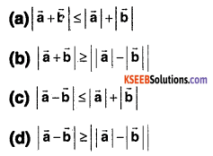When does the equality sign above apply?
a) Geometrical solution:-
Consider the triangle shown in the figure.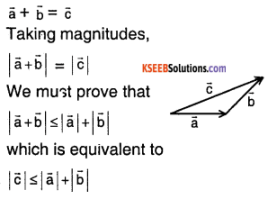which is true by the triangle inequality (Sum of the lengths of any two sides of a triangle is always greater than the length of the third side.)
Equality holds in the case of a degenerate triangle (collinear vectors).
Hence proved.
Analytical solution:-
We must prove that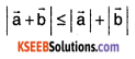sqaure both sides,
To prove that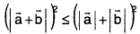i.e., to prove that
a² + b² + 2ab cos θ ≤ a² + b² + 2ab,
( here $$|\overrightarrow{\mathrm{a}}|$$ = a , $$|\overrightarrow{\mathrm{b}}|$$ = b)
i.e., to prove that
2ab cos θ ≤ 2ab i.e., TPT cosθ ≤ 1
Since the range of cose is [-1, 1], the above inequality is true. The equality holds when θ = 0° (collinear vectors).
Hence Proved.

b) Geometrical solution:
Consider the triangle shown in the figure.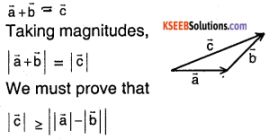Which is true by the triangle inequality. (Difference in the lengths of any two sides of a triangle is always lesser than the length of the third side.) The equality holds for a degenerate triangle (collinear vectors).
Proved.
Analytical solution:-
We are to prove that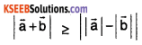Square both sides we must prove that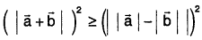i.e., To prove that
a² + b² + 2ab cos θ ≥ a² + b² – 2ab
i.e., to prove that 2ab cos θ ≥ – 2 ab
to prove that cos θ ≥ -1
Since the range of cos θ is [-1, 1], the above inequality is true.
The equality holds when θ = 180° (collinear vectors)
Hence Proved.

c) geometrical solution:-
Consider the triangle shown in the figure.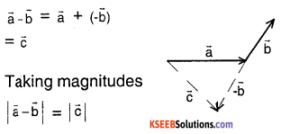we must prove that $$|\overrightarrow{\mathrm{c}}| \leq|\overrightarrow{\mathrm{a}}|+|\overrightarrow{\mathrm{b}}|$$
or to prove that $$|\overrightarrow{\mathrm{c}}| \leq|\overrightarrow{\mathrm{a}}|+|\overrightarrow{\mathrm{- b}}|$$
(Since $$|-\vec{b}|=|\vec{b}|$$) which is true by the triangle inequality. (Sum of the lengths of any two sides of a triangle is always greater than the length of the third side.)
The equality holds when the triangle is degenerate (collinear vectors).
Hence proved.
Analytical solution:-
We must prove that $$|\vec{a}-\vec{b}| \leq|\vec{a}|+|\vec{b}|$$
Square both sides.
To prove that $$(|\vec{a}-\vec{b}|)^{2} \leq(|\vec{a}|+|\vec{b}| |)^{2}$$
i.e., To prove that a² + b² – 2ab cos θ ≤ a² + b² + 2ab
i.e., to prove that -cos θ ≤ 1
Since the range of cos θ is [-1, 1], the range of -cos θ is also [-1, 1].
∴ The above inequality is true. Equality holds when θ = 180° (collinear vectors).
Hence proved.

d) Geometrical solution:-
Consider the triangle shown in the figure.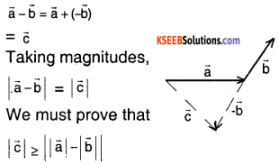i.e., To prove that $$|\vec{c}| \geq|| \vec{a}|-|-\vec{b}||$$
(Since $$|-\vec{b}|=|\vec{b}|$$)
Which is true by the triangle inequality. (Difference in the lengths of any two sides of a triangle is always lesser than the length of the third side.)
The equality holds in the case of a degenerate triangle (collinear vectors).
Hence proved.
Analytical solution:-
We must prove that $$|\vec{a}-\vec{b}| \geq|| \vec{a}|-| \vec{b}||$$
Square both sides.
To prove that $$(|\vec{a}-\vec{b}|)^{2} \geq(|| \vec{a}|-| \vec{b}||)^{2}$$
i.e., To prove that a² + b² – 2ab cose ≥ a² + b² – 2ab
i.e., to prove that cos θ ≤ 1
Since the range of cos θ is [-1, 1],
∴ The above inequality is true.
Equality holds when θ= 0 (collinear vectors).
Hence proved.Question 7.
Given $$\vec{a}+\vec{b}+\vec{c}+\vec{d}=\vec{0}$$. which of the
following statements are correct:
(a) $$\vec{a}, \vec{b}, \vec{c}$$ and $$\vec{d}$$ must each be a null vector,

(b) The magnitude of $$(\vec{a}+\vec{c})$$ equals the magnitude of $$(\vec{b}+\vec{d})$$,

(c) The magnitude of $$\vec{a}$$ can never be greater than the sum of the magnitudes of $$\vec{b}, \vec{c}$$ and $$\vec{d}$$

(d) $$\vec{b} + \vec{c}$$ must lie in the plane of $$\vec{a}$$ and $$\vec{d}$$ if $$\vec{a}$$ and $$\vec{d}$$ if they are not collinear, and in the line of $$\vec{a}$$ and $$\vec{d}$$, if they are collinear?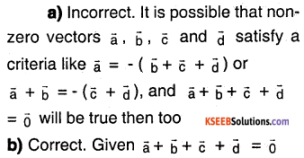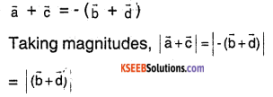c) Correct. By an extension of the triangle inequality (sum of the lengths of any (n-1) sides of an n – sided closed polygon is always greater than the length of the remaining side.)

d) Correct. Given $$\vec{a}+\vec{b}+\vec{c}+\vec{d}=\vec{0}$$
⇒ $$(\vec{b}+\vec{c}) = -(\vec{a}+\vec{d})$$
If $$\vec{a}$$ and $$\vec{d}$$ are not collinear, then $$(\vec{a}+\vec{d})$$ defines a plane. Clearly $$(\vec{b}+\vec{c})$$ has to lie in this plane for the given condition to hold good. Now if $$\vec{a}$$ and $$\vec{d}$$ are collinear, $$\vec{a}$$ + $$\vec{d}$$ is a line and clearly $$(\vec{b}+\vec{c})$$ must be collinear with this line for the given condition to hold good.

Question 8.
Three girls skating on a circular ice ground of radius 200 m start from a point P on the edge of the ground and reach a point Q diametrically opposite to P following different paths as shown in Fig. 4.20. What is the magnitude of the displacement vector for each? For which girl is this equal to the actual length of path skate?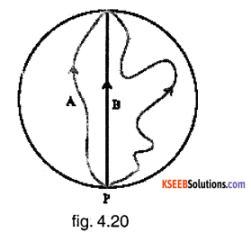The magnitude of the displacement vector for each girl = Shortest distance between points P and Q = Diameter PQ
= 2 × 200 m = 400 m
Since girl B skates along the diameter PQ, for her, the magnitude of displacement vector is equal to the actual length of path skated.

Question 9.
A cyclist starts from the centre O of a circular park of radius 1 km, reaches the edge P of the park, then cycles along the circumference, and returns to the centre along QO as shown in Fig. 4.21. If the round trip takes 10 min, what is the (a) net displacement, (b) average velocity, and (c) average speed of the cyclist?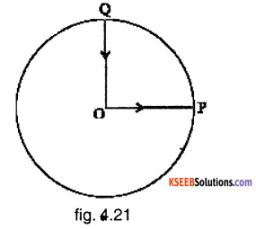a) Since the cyclist returns to the starting point, his net displacement is zero.
b) The average velocity
= $$\frac{\text { Net displacement }}{\text { Time taken }}$$ = 0
c) Average speed = $$\frac{\text { length of the path }}{\text { Time taken }}$$
= $$\frac{\mathrm{OP}+\mathrm{arc} \mathrm{PQ}+\mathrm{QO}}{10 \mathrm{min}}$$
= \frac{1 \mathrm{km}+1/{4} \times 2 \pi \times 1 \mathrm{km}+1 \mathrm{km}}{1 / 6 \mathrm{hour}}[/latex] = 21.4 km / hour.

Question 10.
On open ground, a motorist follows a track that turns to his left by an angle of 60° after every 500 m. Starting from a given turn, specify the displacement of the motorist at the third, sixth and eighth turn. Compare the magnitude of the displacement with the total path length covered by the motorist in each case.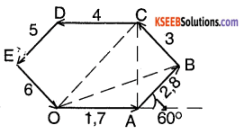Let the origin be O. The path of the motionist is as shown in the figure.
a) After 3 rd turn:-
In triangle ABC, AC = AB cos (∠ACB) + BC cos (∠BAC)
∠ACB = ∠BAC = 30° (Since AB = BC and ∠ABC = 120°).
∴ AC = 500 cos 30° + 500 cos 30°
AC = 866 m
In triangle OAC,
OC = $$\sqrt{\mathrm{OA}^{2}+\mathrm{AC}^{2}}$$ (Since ∠OAC – 90°)
= $$\sqrt{500^{2}+866^{2}}$$ = 1000 m
∴ Magnitude of the displacement = 1 km
Angle with the original direction
= tan-1 $$\left(\frac{\mathrm{AC}}{\mathrm{OA}}\right)$$ = tan-1 $$\left(\frac{866}{500}\right)$$ = 60°
Path length = 3 × 500 m = 1500 m Clearly magnitude of displacement < path length b) After sixth turn:- The net displacement here = 0 (because the motionist returns to his starting point) Angle with the original direction = 0° Path length = 6 × 500 m = 3 km Clearly, path length > magnitude of the displacement.

c) After eighth turn:-
In triangle OAB,
OB = $$\sqrt{\mathrm{OA}^{2}+\mathrm{AB}^{2}+2 . \mathrm{OA} . \mathrm{AB} . \cos \theta}$$
= $$\sqrt{500^{2}+500^{2}+2 \times 500 \times 500 \times \cos 60^{\circ}}$$
= 866 m
Net displacement = 866 m
Angle with the original direction
= $$\tan ^{-1}\left(\frac{A B \sin \theta}{O A+A B \cos \theta}\right)$$
= $$\tan ^{-1}\left(\frac{500 \sin 60^{\circ}}{500+500 \cos 60^{\circ}}\right)$$ = $$\tan ^{-1}\left(1/{\sqrt{3}}\right)$$
= 30°
Path length = 8 × 500 m = 4 km Clearly, path length > Magnitude of net displacement.

Question 11.
A passenger arriving in a new town wishes to go from the station to a hotel located 10 km away on a straight road from the station. A dishonest cab man takes him along a circuitous path 23 km long and reaches the hotel in 28 min. What is

1. the average speed of the taxi,
2. the magnitude of average velocity?
3. Are the two equal?

1. Average speed of the taxi
= $$\frac{\text { Path length }}{\text { Time taken }}$$
= $$\frac{23 \mathrm{km}}{28 \mathrm{min}}$$ = $$\frac{23 \mathrm{km}}{28 \mathrm{28}_{60} \mathrm{h}}$$ = 49.3 km h-1

2. Magnitude of Average Velocity
= $$\frac{\text { Net displacement }}{\text { Time taken }}$$
= $$\frac{10 \mathrm{km}}{28 \mathrm{min}}$$ = $$\frac{10 \mathrm{km}}{28 \mathrm{28}_{60} \mathrm{h}}$$ = 21.4 km h-1

3. Clearly, average speed is greater than magnitude of average velocity. The two are not equal.Question 12.
Rain is falling vertically with a speed of 30 ms-1. A woman rides a bicycle with a speed of 10 ms-1 in the north to south direction. What is the direction in which she should hold her umbrella?
The direction of θ is given by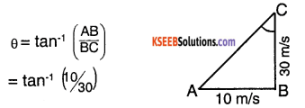= 18° with the vertical.

Question 13.
A man can swim with a speed of 4.0 km/h in still water. How long does he take to cross a river 1.0 km wide if the river flows steadily at 3.0 km/h and he makes his strokes normal to the river current? How far down the river does he go when he reaches the other bank?
Time taken to cross the river
= $$\frac{\text { Width of the river }}{\text { Speed in this direction }}$$
= $$\frac{1 \mathrm{km}}{4 \mathrm{km} \mathrm{h}^{-1}}$$ = $$1 / 4$$ h = 15 min
Distance along the river covered in this time
= Speed of the river x Time taken to cross the river
= 3 km h-1 × 15 min = 0.75 km.

Question 14.
In a harbour, wind Is blowing at the speed of 72 km/h and the flag on the mast of a boat anchored in the harbour flutters along the N-E direction. If the boat starts moving at a speed of 51 km/ h to the north, what is the direction of the flag on the mast of the boat?
Answer: When the boat moves, the flag experiences wind blowing at 51 km h-1 in the south direction. Let the flag be at an angle 0 with the East direction.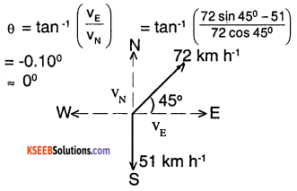∴ The flag blows along the east direction.

Question 15.
The ceiling of a long hall is 25 m high. What Is the maximum horizontal distance, that a ball thrown with a speed of 40 m s-1 can go without hitting the ceiling of the hall?
Given hm = 25 m , g = 9.8 ms-2 v0 = 40 ms-1
hm = $$\frac{\left(v_{0} \sin \theta_{0}\right)^{2}}{2 g}$$
25 = $$\frac{\left(40 \sin \theta_{0}\right)^{2}}{2 \times 9.8}$$
i.e. (40 sin θ0)² = 490
⇒ 40 sin θ0 = $$7 \sqrt{10}$$
⇒ sin θ0 = $$\frac{7 \sqrt{10}}{40}$$
⇒ θ0 = 33.6°
Maximum horizontal distance
R = $$\frac{v_{0}^{2}}{g}$$ Sin 2 θ = $$\frac{40^{2}}{9.8}$$sin(2 × 33.6°)
= 150.5 m

Question 16.
A cricketer can throw a ball to a maximum horizontal distance of 100 m. How much high above the ground can the cricketer throw the same ball?
Given R = $$\frac{v_{0}^{2}}{g}$$ sin 2 θ = 100 m
Since R is maximum, θ = 45°
R = $$\frac{v_{0}^{2}}{g}$$ = 100 m
Maximum height is achieved when throwing angle is 90°.
hmax = $$\frac{\left(v_{0} \sin 90^{\circ}\right)^{2}}{29}$$
= $$\frac{v_{0}^{2}}{g}$$ = $$\frac{1}{2} \times \frac{v_{0}^{2}}{g}$$
1/2 × 100 m = 50 m.
∴ Maximum height hmax = 50m.

Question 17.
A stone tied to the end of a string 80 cm long is whirled in a horizontal circle with a constant speed. If the stone makes 14 revolutions in 25 s, what is the magnitude and direction of acceleration of the stone?
Radius of the circle r = 80 cm = 0.8m Angular speed
υ = $$\frac{14 \times 2 \pi r}{t}$$
υ = $$\frac{14 \times 2 \times 22 / 7 \times 0.8}{25}$$
υ = 2.816 ms-1
Acceleration = $$\frac{v^{2}}{r}$$ = $$\frac{2.816^{2}}{0.8}$$
= 9.9 ms-2
Direction of acceleration: Towards the centre of the circle and along the radius.

Question 18.
An aircraft executes a horizontal loop of radius 1.00 km with a steady speed of 900 km/h. Compare its centripetal acceleration with the acceleration due to gravity.
Radius r = 1.00 km = 1000 m
Speed v = 900 km h-1 = 250 ms-1
Centripetal acceleration
a = $$\frac{v^{2}}{r}=\frac{250^{2}}{1000}$$
a = 62.5 ms-2
Acceleration due to gravity g = 9.8 ms-2.
a = $$\frac{62.5}{9.8}$$ = 6.4 g

question 19.
Read each statement below carefully and state, with reasons, if it is true or false:

1. The net acceleration of a particle in circular motion is always along the radius of the circle towards the centre
2. The velocity vector of a particle at a point is always along the tangent to the path of the particle at that point
3. The acceleration vector of a particle in uniform circular motion averaged over one cycle is a null vector

1. False. The statement is true only if the particle is executing circular motion at a uniform speed (no tangential acceleration).
2. True. The instantaneous velocity is in the direction tangential to the instantaneous path of the particle.
3. True. Consider the two points and on the circle as shown in the figure.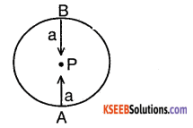At both these points, acceleration is towards the centre P. The net acceleration is O (equal magnitude but of opposite directions). Similarly, over one cycle, the net acceleration is a null vector.

Question 20.
The position of a particle is given by
$$r=3.0 t \hat{i}-2.0 t^{2} \hat{j}+4.0 \hat{k} m$$
where t is in seconds and the coefficients have the proper units for r to be in meters.

1. Find the v and an of the particle?
2. What is the magnitude and direction of velocity of the particle at t = 2.0 s?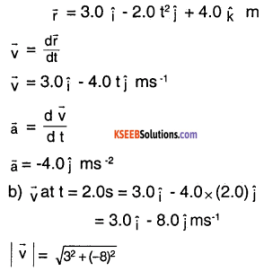= 8.54 ms-1
Direction θ = $$\tan ^{-1}\left(\frac{v_{y}}{v_{x}}\right)$$
= $$\tan ^{-1}\left(\frac{-8}{3}\right)$$
= – 69.44° with the horizontal (x – axis)Question 21.
A particle starts from the origin at t = 0 s with a velocity of 10.0 J m/s and moves in the x-y plane with a constant acceleration of (8.0 I + 2.0 J) m s-2.

1. At what time is the x – coordinate of the particle 16 m? What is the y – coordinate of the particle at that time?
2. What is the speed of the particle at the time?

1. Along x – direction,
vx(0) = 0, ax = 8 ms-22
rx(t) = rx(0) + vx(o) t + 1/2 ax
= 0 + 0 + 1/2 × 8 × t² = 4 t²
Given rx(t1) = 16 m
4 t² = 16m
⇒ t = 2 s
∴ At time t = 2.0 s, x – coordinate of the particle = 16 m
Along y – direction,
vy(o) = 10 ms-1, ay = 2 ms-2
ry(t) = ry(0) + vy(0)t +1/2 ay
0 + 10 t + 1/2 × 2 × t²
= 10 t + t²
ry(2) = 10(2) + (2)² = 24 m
∴ y – coordinate at time t = 2.0 s is 24 m

2. Along x – direction,
Vx(t) = vx (0) + axt
= 0 + 8t = 8t
vx(2) = 16 ms-1
Along y – direction,
vy(t) = vy(0) + ayt
= 10 + 2t
vy (2) = 14 ms-1
Speed at time t a 2.0 s is $$\sqrt{\left(v_{x}(2)\right)^{2}+\left(v_{y}(2)\right)^{2}}$$
= $$\sqrt{16^{2}+14^{2}}$$ = 21.26 ms-1

Question 22.
I and J are unit vectors along x- and y-axis respectively. What is the magnitude and direction of the vectors I+ J, and I – J? What are the components of a vector A = 2I+3J along the directions of I + J and l – j? [You may use graphical method]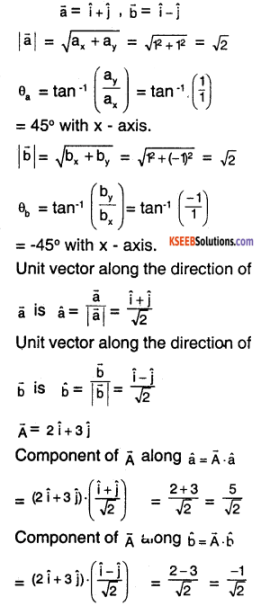Question 23.
For any arbitrary motion in space, which of the following relations are true:

1. vaverage = (1/2) (v (t1 + v (t2)))
2. vaverage = [r(t2) – r(t1)] /(t2 – t2)
3. v (t) = v (0) + a t
4. r (t) = r (0) + v (0) t + (1/2) a t²
5. aaverage = [v(t2) – v(t1)]/(t2 – t1)

(The ‘average’ stands for average of the quantity over the time interval t1 to t2

1. False. Average velocity is not the arithmetic mean of the individual velocities.
2. True. By definition.
3. False. This is true only if the acceleration $$\overrightarrow{\mathrm{a}}$$ is constant.
4. False. This is true only if the acceleration $$\overrightarrow{\mathrm{a}}$$ is constant.
5. True. By definition.

Question 24.
Read each statement below carefully and state, with reasons and examples, if it is true or false:
A scalar quantity is one that

1. is conserved in a process
2. can never take negative values
3. must be dimensionless
4. does not vary from one point to another in space
5. has the same value for observers with different orientations of axes.

1. False. Mass is a scalar quantity and it is not conserved in a nuclear reaction.
2. False. Angle is a scalar quantity and it can take negative values.
3. False. Mass, current, etc. are scalar quantities that have dimensions.
4. False, Temperature, electric potential, etc. are scalar quantities that vary from point to point in a medium.
5. True. Change of axis does not change a scalar quantity. For example, mass is a scalar quantity that is independent of the axis chosen.

Question 25.
An aircraft is flying at a height of 3400 m above the ground if the angle subtended at a ground observation point by the aircraft positions 10.0 s apart is 30°, what is the speed of the aircraft?
Let O be the observation point. A and B are the initial and final positions of the aircraft. Angle substended θ = 30°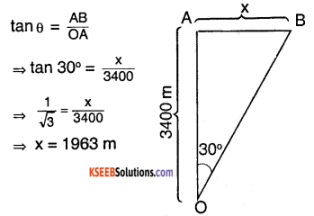= $$\frac{\text { Distance coverd }}{\text { Time taken }}=\frac{1960 \mathrm{m}}{10 \mathrm{s}}$$ = 196 ms-1.

### 1st PUC Physics Motion in a Plane Additional Exercises Questions and Answers

Question 26.
A vector has magnitude and direction. Does it have a location in space? Can it vary with time Will two equal vectors a and b at different locations in space necessarily have Identical physical effects? Give examples in support of your answer.
Yes, a vector has a defined location in space. It may or may not vary with time. For example, $$3 \hat{i}+4 \hat{j}$$ is time independent whereas $$4 t \hat{i}-7 \sqrt{t} \hat{k}$$ is time dependent.
Two vectors at different locations need not have the same physical effects. Consider the weight of a person in newtons, ‘k’ newtons of weight have different meanings on earth and on the moon, and so they have different physical effects.

Question 27.
A vector has both magnitude and direction. Does it mean that anything that has magnitude and direction is necessarily a vector? The rotation of a body can be specified by the direction of the axis of rotation, and the angle of rotation about the axis. Does that make any rotation a vector?
A quantity that has magnitude and direction need not necessarily be a vector. Consider finite rotations of a particle about an axis. 2 complete rotations bring back the particle to its initial position but these rotations do not add up as per. Parallelogram law of addition. Hence, rotation is not a vector.

Question 28.
Can you associate vectors with

1. the length of a wire bent into a loop,
2. a plane area,
3. a sphere? Explain.

1. No.
2. Yes. The area $$\overrightarrow{\mathrm{A}}$$ can be defined as $$\overrightarrow{\mathrm{A}}$$ = $$\overrightarrow{I} \times \overrightarrow{\mathbf{b}}$$ where $$\overrightarrow{i}$$ and $$\overrightarrow{b}$$ are the length and breadth vbctors.
3. No.Question 29.
A bullet fired at an angle of 30° with the horizontal hits the ground 3.0 km away. By adjusting its angle of projection, can one hope to hit a target 5.0 km away? Assume the muzzle speed to the fixed, and neglect air resistance.
Let ‘u’ be the muzzle speed.
0=30°
Range R = $$\frac{\mathfrak{u}^{2} \sin 2 \mathfrak{\theta}}{\mathfrak{g}}$$
3000 m = $$\frac{\mathrm{u}^{2}}{\mathrm{g}}$$ × sin (2 × 30°)
⇒ $$\frac{\mathrm{u}^{2}}{\mathrm{g}}$$ = $$\frac{3000}{\sin 60^{\circ}}$$ = 3464 m.
Maximum range possible Rmax = $$\frac{\mathrm{u}^{2}}{\mathrm{g}}$$
= 3.46 km
∴ It is not possible to hit a target 5 km away.

Question 30.
A fighter plane flying horizontally at an altitude of 1.5 km with speed 720 km/h passes directly overhead an antiaircraft gun. At what angle from the vertical should the gun be fired for the shell with muzzle speed 600 m s-1 to hit the plane? At what minimum altitude should the pilot fly the plane to avoid being hit?
(Take g = 10 m s-2).
Plane’s altitude
h = 1.5 km = 1500 m
Plane’s speed
Vp = 720 km/h = 200 ms-1
Shell’s speed
Vs = 600 ms-1
Let the shell be fired at an angle θ with the vertical.
Horizontal distance covered by the shell in time t = Horizontal distance covered by the plane in time t
⇒ Vs sin θ .t = Vpt
⇒ 600 sin θ = 200
⇒ θ = sin-1(1/3) = 19.5°
Minimum altitude to ensure no hit = Maximum height achieved by the shell
= $$\frac{\mathrm{u}^{2} \cos ^{2} \theta}{2 \mathrm{g}}$$
= $$\frac{600^{2} \cos ^{2}\left(19.5^{\circ}\right)}{2 \times 10}$$
= 1600 m
= 16 km.

Question 31.
A cyclist is riding with a speed of 27 km/h. As he approaches a circular turn on the road of radius 80 m, he applies brakes and reduces his speed at the constant rate of 0.50 m/s every second. What is the magnitude and direction of the net acceleration of the cyclist on the circular turn?
Speed of the cyclist v = 27 km/h
= 7.5 ms-1.
Radius of the turn r = 80 m
Centripetal acceleration ac = $$\frac{v^{2}}{r}=\frac{7.5^{2}}{80}$$
= 0.70 ms -2
Tangential retardation ar = $$\frac{0.5}{1}$$ = 0.5 ms-2
Net acceleration a = $$\sqrt{a_{c}^{2}+a_{r}^{2}}$$
= $$\sqrt{0.70^{2}+0.5^{2}}$$
= 0.86 ms-2
Angle θ with the tangent is given by
θ = tan-1 $$\left(\frac{\mathrm{a}_{\mathrm{r}}}{\mathrm{a}_{\mathrm{t}}}\right)$$
θ = tan-1$$\left(\frac{0.70}{0.50}\right)$$
θ = 54.5°.

Question 32.
(a) Show that for a projectile the angle between the velocity and the x-axis as a function of time is given by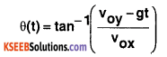(b) Shows that the projection angle θ for a projectile launched from the origin is given by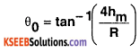where the symbols have their usual meaning.
(a) Let the horizontal component of the initial velocity be vox and the vertical component be voy.
During motion, the horizontal component is still vox but the vertical component
= Voy – gt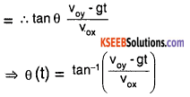(b) For the projectile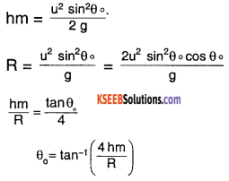### 1st PUC Physics Motion in a Plane One Mark Questions and Answers

Question 1.
What is the work done by a body in circular motion?
Zero.

Question 2.
What is the trajectory of a projectile?
Parabola.

Question 3.
What is velocity of projection?
The velocity with which the body is projected is called velocity of projection.

Question 4.
What is angle of projection?
The angle between the direction of projection and the horizontal drawn at that point is called the angle of projection.

Question 5.
What is range of projectile?
The maximum horizontal distance covered by the projectile is called the range.

Question 6.
What is time of flight?
The time taken to describe the range is called the time of flight.

Question 7.
When is the range of a projectile is maximum?
When the angle of projection is 45° the range of a projectile is maximum.

Question 8.
Why should an athlete throw the javelin or shot put approximately at an angle of 45°?
An athlete must throw shot – put or a javelin approximately at an angle 45°to achieve the maximum range.

Question 9.
What is uniform circular motion?
Motion of a body along a circular path with constant speed is called uniform circular motion.Question 10.
What is the direction of motion (or velocity) of a body in uniform circular motion?
The direction of motion of the body at any instant will be along the tangent to the circular path.

Question 11.
Define angular displacement.
The angle through which the radius vector rotates is called angular displacement.

Question 12.
Define angular velocity.
The rate of angular displacement is called angular velocity.

Question 13.
Define period of revolution of a body.
It is the time taken by the body to complete one revolution.

Question 14.
Define frequency of revolution of a body.
Number of revolutions completed by the body in one second is called frequency of revolution.

Question 15.
Define centripetal acceleration.
In uniform circular motion, the acceleration produced on the body acts along the radius, towards the centre of the circular path and is known as centripetal acceleration or radial acceleration.

Question 16.
What is the source of centripetal force for the planets revolving round the sun?
The gravitational force of attraction between the sun and the planets provides the centripetal force for the revolution of the planets round the sun.

Question 17.
Raising the outer edge of the road to get the required centripetal force for a vehicle is known as banking of roads (or track).

Question 18.
What is the work done by centripetal force?

Question 19.
A body moves along a circle of radius 3m. What is the distance travelled. When it completes one circle?
Distance travelled by. the body when it completes one circle = circumference of the circle
= 2 π r = 2 × 3.4 × 3
= 18.84 m

Question 20.
Give an example for three dimen-sional motion.
Flight of Aeroplane or movement of gas molecule in space.

Question 21.
Give an example of centripetal force.
Electrostatic force between an electron and nucleus provides centripetal force for the revolution of the electron in its orbit.

Question 22.
Which vector quantity becomes zero at highest point of motion of projectile?
Vertical component of velocity becomes zero at the highest point.

Question 23.
State the law of parallelogram of two forces.
If two forces acting at a point are represented both in magnitude and direction by the two adjacent sides of a parallelogram then their resultant is represented by the diagonal of the parallelogram drawn from the same point.

Question 24.
What will be the effect on the horizontal range of a projectile when its initial speed is doubled keeping the angle the same?
Since R α v0², doubling the initial speed will increase the range by a factor of 2² = 4 times.Question 25.
What is the minimum number of forces acting on a object in a plane that can produce a zero resultant force?
Two. (The forces should have equal magnitude but opposite direction).

Question 26.
A unit vector Is represented by $$\mathbf{a} \hat{\mathbf{i}}+\mathbf{b} \hat{\mathbf{j}}+\mathbf{c} \hat{\mathbf{k}}$$. If the values of a and b are
0.6 and 0.8 respectively, find the value of C.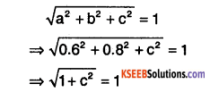⇒ c = 0

### 1st PUC Physics Motion in a Plane Two Mark Questions and Answers

Question 1.
When is a body said to have two-dimensional motion? Give an example.
Motion of a particle in a plane is known as two-dimensional motion. Example:

• A car moving along a zig-zag path on a road.
• Motion of the planet around the sun in its orbit.

Question 2.
What is a projectile? Give an example.
Anybody was thrown into space, that moves under the effect of gravity is called a projectile.
E.g.:

• A bullet fired from the gun.
• A javelin thrown by an athlete.

Question 3.
Obtain the relation between angular velocity and linear velocity.
Consider a particle moving in a circle of radius ‘r’ with uniform angular velocity ‘ ω ’ and linear speed ‘V’. Let the particle moves from A to B in time ‘t’ seconds through a small distance ‘s’ on the circumference. Let θ be the angular displacement.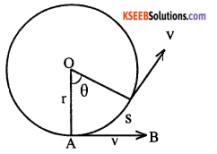Angular velocity ω = $$\frac{\theta}{\mathfrak{t}}$$
Angular displacement θ = $$\frac{s}{\mathfrak{r}}$$
∴ ω = $$\frac{\mathrm{s}}{\mathrm{tr}}$$
But $$\frac{s}{\mathfrak{t}}$$ = v, linear velocity
∴ ω = $$\frac{\mathrm{v}}{\mathrm{r}}$$ or
v = r ω
linear velocity = radius × angular velocity.

Question 4.
What is angle of banking? Give the expression for it.
The angle through which the outer edge of the roads are raised is called the angle of banking. The angle of banking is given by,
θ = tan-1$$\left(\frac{v^{2}}{r g}\right)$$
where m is the mass, v is the velocity and r is the radius.

Question 5.
A body travels one round in a circle of radius ‘R’- What is the

• displacement and.
• distance traveled.

• The displacement of a body = 0
• The distance travelled = 2nR

Question 6.
Write the expressions for the maximum height reached by a projectile, and explain the terms.
The expression for the maximum height reached by the projectile is
$$\mathrm{H}=\frac{u^{2} \sin ^{2} \theta}{2 \mathrm{g}}$$
Where u, is the initial velocity of the projectile, θ is the angle between the horizontal & the initial velocity, g is the acceleration due to gravity.

Question 7.
What is banking of roads? Give an example of it.
Vehicles while taking a turn cannot incline themselves to one side to get the required centripetal force. To provide necessary centripetal force without slipping. The outer edge of the road is raised over the inner edge.

Question 8.
Why a cyclist bends inward when to Is crossing a curve in a road?
He bends inward to get sufficient centripetal force.Question 9.
Two equal forces have their resultant equal to either. What is the inclination between them?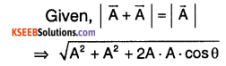= A
⇒ 2A² (1 + cos θ) = A²
⇒ 1 + cos θ = 1/2
⇒ cos θ = -1/2
⇒ θ = 120°

Question 10.
A cyclist has to bend a little Inwards from his vertical position while turning. Why?
Bending provides a component of the normal reaction force from the ground to provide the cyclist the necessary centripetal force for turning. Hence, bending is necessary.

Question 11.
Suppose you have two forces $$\overrightarrow{\mathrm{F}}$$ and $$\overrightarrow{\mathrm{F}}$$. How would you combine them in order to have resultant forces of magnitudes

1. zero
2. 2 $$\overrightarrow{\mathrm{F}}$$, and
3. $$\overrightarrow{\mathrm{F}}$$?

1. If the forces act in opposite directions, the resultant is zero.
2. If the forces act in the same direction, resultant $$\overrightarrow{\mathrm{R}}$$ = 2 $$\overrightarrow{\mathrm{F}}$$.
3. For $$\overrightarrow{\mathrm{R}}$$ = 2 $$\overrightarrow{\mathrm{F}}$$
$$\sqrt{\mathrm{F}^{2}+\mathrm{F}^{2}+2 \mathrm{F} \cdot \mathrm{F} \cdot \cos \theta}$$ = F
⇒ 2F² (1 + cos θ) = F²
⇒ 1 + cos θ = 1/2
⇒ θ = 120°

Question 12.
Prove the following statement: “For elevation which exceeds or falls short of 45° by equal amounts, the range Is equal”.
At 45° – θ, the range is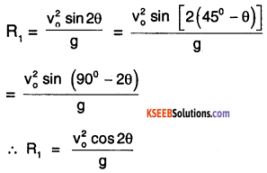At 45° + θ, the range is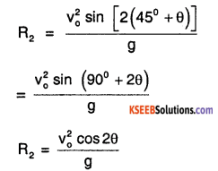⇒ R2 = R1 Proved.

Question 13.
What is the distance traveled by a point during time ‘t’, if it moves in x – y plane according to the relation x = a sin cot and y = a(1 – cos cot ωt)?
Distance travelled d(t) =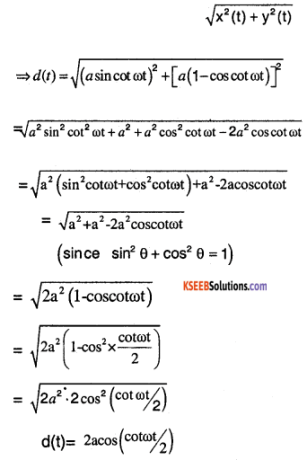Question 14.
A lady walking due east on a road with velocity 10 ms-1 encounters rain falling vertically with a velocity of 30ms-1. At what angle should she hold her umbrella to protect herself from the rain?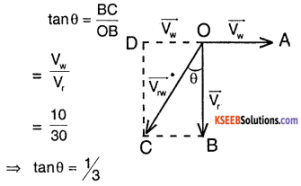⇒ θ = 18°16′ with the vertical direction.

Question 15.
If the vectors of equal magnitude add to either of them by magnitude, what is the angle between them?

1. If the forces act in opposite directions, the resultant is zero.
2. If the forces act in the same direction, resultant $$\overrightarrow{\mathrm{R}}$$ = 2 $$\overrightarrow{\mathrm{F}}$$.
3. For $$\overrightarrow{\mathrm{R}}$$ = 2 $$\overrightarrow{\mathrm{F}}$$
$$\sqrt{\mathrm{F}^{2}+\mathrm{F}^{2}+2 \mathrm{F} \cdot \mathrm{F} \cdot \cos \theta}$$ = F
⇒ 2F² (1 + cos θ) = F²
⇒ 1 + cos θ = 1/2
⇒ θ = 120°

Question 16.
A swimmer can swim with velocity of 10km h-1 w.r.t. the water flowing in a river with velocity of 5 km h-1. In what direction should he swim to reach the
point on the other bank just opposite to his starting point?
Let the angle of the swimmer be e w.r.t the horizontal.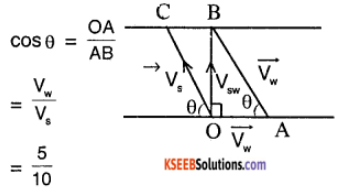⇒ cos θ = 1/2
⇒ θ = 60°

Question 17.
The angle between vector $$\overrightarrow{\mathbf{A}}$$ and \overrightarrow{\mathbf{B}} is 60°. What is the ratio of $$\overrightarrow{\mathbf{A}} \cdot \overrightarrow{\mathbf{B}}[latex] and [latex]|\overrightarrow{\mathbf{A}} \times \overrightarrow{\mathbf{B}}|[latex]? Answer: Required ratio [latex]=\frac{\overrightarrow{\mathrm{A}} \cdot \overrightarrow{\mathrm{B}}}{\overrightarrow{\mathrm{A}} \times \overrightarrow{\mathrm{B}}}$$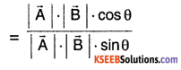= cot θ
= cot (60°)
= $$1 / \sqrt{3}$$

Question 18.
Two forces P=10 N and Q = 15 N are acting at a point making an angle 30° with each other. What is the cross product of P
and Q?
P= 10N, Q = 15N, θ =30°
From the equation P × Q = P.QSine
= 10.15 Sin 30° = 75N.

### 1st PUC Physics Motion in a Plane Three Mark Questions and Answers

Question 1.
Find a unit vector parallel to the vector $$3 \hat{i}+7 \hat{j}+4 \hat{k}$$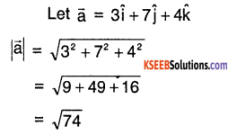Unit vector parallel to $$\overrightarrow{\mathrm{a}}=\frac{\overrightarrow{\mathrm{a}}}{|\overrightarrow{\mathrm{a}}|}$$
$$=\frac{3 \hat{i}+7 \hat{j}+4 \hat{k}}{\sqrt{74}}$$

Question 2.
What is a projectile? Show that the path followed by an oblique projectile Is a parabola.
Anybody was thrown into space, that moves under the effect of gravity is called a projectile.
E.g.:
1. A bullet fired from the gun.
2. A javelin thrown by an athlete., Consider a particle thrown up at an angle θ to the horizontal with a velocity u. The velocity of projection can be resolved into two component u cos θ and usinq along OX and OY respectively.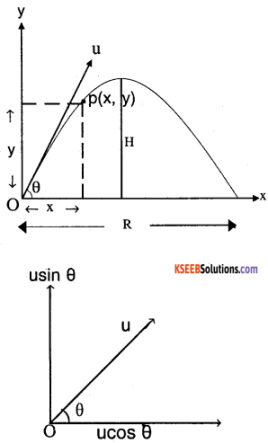The horizontal component remains constant while the vertical component is affected by gravity.
Let P(x, y) be the position of the projectile after ‘t’ seconds.
Then distaince travelled ‘x’ along OX is given by,
x = horizontal component of the velocity time
x = u cos θ × t ………… (1)
The distance travelled along the vertical direction in same time‘t’ is,
y = (u sin θ) t – 1/2 gt² ……………… (2)
Substituting, t = $$\frac{x}{u \cos \theta}$$ from (1) in (2),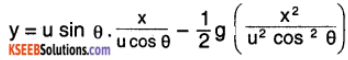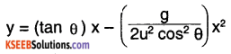…………………..(3)
where a = tan θ, $$\mathrm{b}=\frac{\mathrm{y}=\mathrm{gax}-\mathrm{bx}^{2}}{2 \mathrm{u}^{2} \cos ^{2} \mathrm{\theta}}$$ are constant for given values of θ, u and g.
The equation (3) represents a parabola. Therefore trajectory of a projectile is a parabola.

Question 3.
Establish a relation between linear velocity and angular velocity in a uniform circular motion and explain the direction of the velocity.
The distance ‘s’ covered by a body travelling in an arc of radius Y and turning its radial line by ‘θ’ is given by
s = r θ
Differentiating both sides w.r.t. time, we have
$$\frac{\mathrm{ds}}{\mathrm{dt}}=\mathrm{r} \frac{\mathrm{d} \theta}{\mathrm{dt}}$$
i.e., v = rw
or Linear velocity = radius × angular velocity.
At each point the body moves along the tangent.
The presence of centripetal force
F =$$\frac{m v^{2}}{r}$$ makes the body to travel in the circular path.
Thus, the direction of velocity is always along the tangent at any point in the circular path.

Question 4.
Find the magnitude and direction of the resultant of two forces p and Q in terms of their magnitudes and angle Q between them.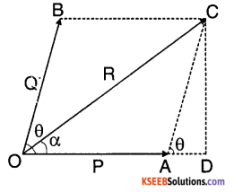P and Q are two forces acting at a point O; making an angle θ. They are represented by OA and OB. the diagonal OC of the parallelogram OBCA represents the resultant R.
Draw CD perpendicular to extended line of OA. In the right angle triangle ODC.
OC² = OD² + CD²
= (OA + AD)² + CD²
= OA² + 2OA. AC cos θ + AC²
R² = P² + 2PQcos θ + Q² v
AC² = AD² + CD² & $$\frac{\mathrm{AD}}{\mathrm{AC}}$$ = cosq
Magnitude of the resultant
R = $$\sqrt{\mathrm{P}^{2}+\mathrm{Q}^{2}+2 \mathrm{PQ} \cdot \cos \theta}$$
To find the direction:
If a is the angle made by R with P, then from the right angled triangle ODC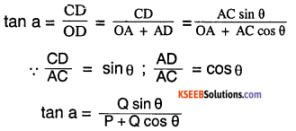Question 5.
In long jump, does it matter how high you jump? What factors determine the span of the jump?
In order to increase the span of the jump, it is necessary for the horizontal component of the velocity to be more than the vertical component. The height of the jump does not matter.
The factors that determine the span of the jump are:

1. angle of elevation at the time of jumping.
2. Speed of the runner at the time of jumping.

Question 6.
Determine a unit vector which Is per-pendicular to both $$\vec{A}=2 \hat{i}+\hat{j}+\hat{k}$$ and $$\overrightarrow{\mathbf{B}}=\hat{\mathbf{i}}-\hat{\mathbf{j}}+2 \hat{\mathbf{k}}$$
The unit vector $$\hat{n}$$ is given by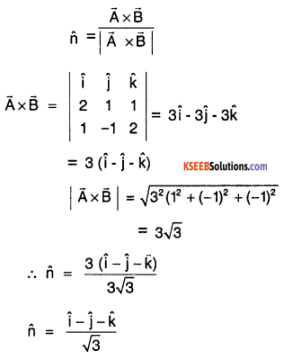Question 7.
An accelerating train is passing over a high bridge. A stone is dropped from the train at an instant when Its speed is 10 ms-1 and acceleration is 1ms-2. Find the horizontal and vertical components of the velocity and acceleration of the stone one second after it is dropped.Take g = 10 ms-2.
Horizontal acceleration = 0 (because there is no force acting on the stone in the horizontal direction to provide any acceleration)
Horizontal velocity = 10 ms-1.
Vertical acceleration = g = 10ms-2.
Vertical velocity = ut + 1/2 gt²
= 0(1) + 1/2 (10)(1)²
= 5 ms-1.

Question 8.
A projectile is projected with velocity ‘u’ making an angle θ with the horizontal direction. Find:

1. Time of flight
2. Horizontal range

1. Let H be the maximum height reached by the projectile in time t1
For vertical motion,
The initial velocity = usin θ
The final velocity = 0
Acceleration = – g
using, v² = u² + 2as
0 = u² sin2 θ – 2gH
2gH =u²sin2 θ
u² sin² θ
H = $$\frac{\mathrm{u}^{2} \sin ^{2} \theta}{2 \mathrm{g}}$$

2. Let t, be the time taken by the projectile to reach the maximum height H.
For vertical motion,
initial velocity =usin0
Final velocity at the maximum height = 0
Acceleration a = – g
Using the equation v = u + at1
0 = usin θ – gt1
gt1 = usin θ
t1 = $$\frac{\mathrm{usin} \theta}{\mathrm{g}}$$
Let t2 be the time of descent.
But t1 = t2
i.e. time of ascent = time of descent.
Time of flight T = t1 + t2 = 2t1
∴ T = $$\frac{2 u \sin \theta}{g}$$

Question 9.

1. Show that for two complementary angles of projection of a projectile with the same velocity, the horizontal ranges are equal.
2. For what angle of projectile is the rangle maximum?
3. For what angle of projection of a projectile are the horizontal range and height attained by the projectile equal?

1. For projection angle θ,
R1 = $$\frac{u^{2} \sin 2 \theta}{g}$$
For projection angle 90° – θ,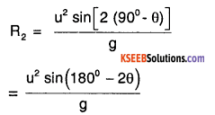But sin (180° – 2θ) = sin2θ
∴ R2 = $$\frac{\mathrm{u}^{2} \sin 2 \theta}{\mathrm{g}}$$ = R1

2. Range is maximum when projection angle = 45°.

3. Given R = H
⇒ $$\frac{\mathrm{u}^{2} \sin 2 \theta}{\mathrm{g}}$$ = $$\frac{\mathrm{u}^{2} \sin ^{2} \theta}{2 \mathrm{g}}$$
⇒ 2 sin θ cos θ = $$\frac{\sin ^{2} \theta}{2}$$
⇒ cot θ = 1/4
θ = cot-1(1/4)
⇒ θ = 75.96°.Question 10.
What is meant by centripetal acceleration? Derive the formula for centripetal acceleration.
In uniform circular motion, the acceleration produced on the body acts along the radius, towards the centre of the circular path. This acceleration is known as centripetal acceleration or radial acceleration.
Let a particle of mass m be moving around the circle of radius r with a uniform speed ‘v’.
Let the particle moves from A to B in a time ‘t’ seconds covering a small angle θ.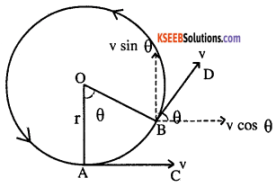At the point A the velocity of the particle is along the tangent AC and therefore there is no component along AO (i.e. along radius).
At the point B the velocity of the particle is along BD. This can be resolved into two components v cos θ and v sin θ. Since θ is small sin θ ≅
θ and cos θ ≅ 1.
∴ change in velocity parallel to AC
= v cos θ – v = v -v = 0. ∵ cos θ ≅ 1.
∴ There is no acceleration along the tangent,
Change in velocity parallel to OA is
= vsin θ – 0 = v θ ( sinθ ≅ θ)
∴ Acceleration along AO = $$\frac{\mathrm{v} \theta}{\mathrm{t}}$$
i.e., centripetal acceleration = $$\frac{\mathrm{v} \theta}{\mathrm{t}}$$ = v ω
∴ centripetal force = mass × centripetal acceleration
F=m v ω
F = mw²r = $$\frac{m v^{2}}{r}$$ ∵v = ω r

Question 11.
From the top of a tower of 100m height, a ball is dropped and at the same time another ball is projected vertically upwards from the ground with a velocity of 25ms-1. Find when and where the two balls will meet. Take g = 9.8 ms-2
Let AC = x
Then BC = 100 – x
x = ut + 1/2 g t²
x = 4.9 t² …………… (1)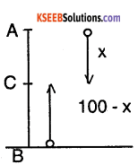BC = ut – 1/2 g t²
= 25 t – 1/2 × 9.8 t²
100 – x = 25 t – 4.9 t² ………………. (2)
Substituting 4.9 t² = x from (1) in (2)
100 – 2 = 25 t – x
⇒ t = 4 seconds
x = 4.9 t²
= 4.9 × 4²
= 78.4 m from the top or 21.6 m from the ground.

### 1st PUC Physics Motion in a Plane FourFive Marks Questions and Answers

Question 1.
Show that the trajectory of a projectile is a parabola.
Consider a particle thrown up at an angle q to the horizontal with a velocity u. The velocity of projection can be resolved into two component u cos θ and u sin θ along OX and OY respectively.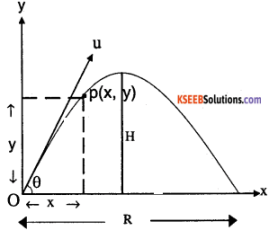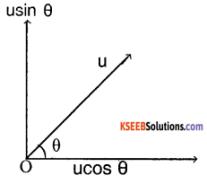The horizontal component remains constant while the vertical component is affected by gravity.
Let P(x, y) be the position of the projectile after ‘t’ seconds.
Then distance travelled ‘x’ along OX is given by,
x = horizontal component of the velocity × time
x = ucos θ × t …………………. (1)
The distance travelled along the vertical direction in same time ‘t’ is,
y = (usin θ) t – 1/2gt² ………… (2)
Substituting, t = $$\frac{x}{u \cos \theta}$$ from (1) in (2),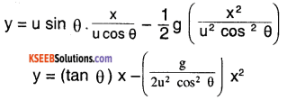y = ax – bx² …………………… (3) where
a = tan θ, $$\mathrm{b}=\frac{\mathrm{y}=\mathrm{gax}-\mathrm{bx}^{2}}{2 \mathrm{u}^{2} \cos ^{2} \mathrm{\theta}}$$, are constants
for given values of θ, u and g.
Equation (3) represents a parabola. Therefore trajectory of a projectile is a parabola.

Question 2.
Derive an expression for the maximum height reached, time of flight and range of a projectile.
(i) Let H be the maximum height reached by the projectile in time t1 For vertical motion,
The initial velocity = usin θ
The final velocity = 0
Acceleration = – g
∴ using, v² = u² + 2as
0 = u²sin² θ – 2gH
2gH = u²sin² θ
H = $$\frac{u^{2} \sin ^{2} \theta}{2 g}$$

(ii) Let t, be the time taken by the projectile to reach the maximum height H.
For vertical motion,
initial velocity = usin θ
Final velocity at the maximum height = 0
Acceleration a = – g
Using the equation v = u + at1
0 = usin θ – gt1
gt1 = usin θ
t1 = $$\frac{\mathrm{usin} \theta}{\mathrm{g}}$$
Let t2 be the time of descent.
But t1 = t2
i.e. time of ascent = time of descent.
∴ Time of flight T = t1 + t2 = 2t1
∴ T = $$\frac{2 u \sin \theta}{\dot{g}}$$

(iii) Let R be the range of the projectile in a time T. This is covered by the projectile with a constant velocity ucos θ.
Range = horizontal component of
velocity × Time of flight
i.e, R = ucos θ. T
R = ucos θ. $$\frac{2 u \sin \theta}{g}$$
R= $$\frac{u^{2} \sin 2 \theta}{g}$$
∵ 2 sin θ.cos θ = sin2 θ.

Question 3.
Derive an expression for the centripetal force.
Let a particle of mass m be moving around the circle of radius r with a uniform speed ‘V’.
Let the particle moves from A to B in a time ‘t’ seconds covering a small angle θ.At the point A the velocity of the particle is along the tangent AC and therefore there is no component along AO (i.e. along radius).
At the point B the velocity of the particle is along BD. This can be resolved into two components v cos θ and v sin θ. Since θ is small sin θ ≅
θ and cos θ ≅ 1.
∴ change in velocity parallel to AC
= v cos θ – v = v -v = 0. ∵ cos θ ≅ 1.
∴ There is no acceleration along the tangent,
Change in velocity parallel to OA is
= vsin θ – 0 = v θ ( sinθ ≅ θ)
∴ Acceleration along AO = $$\frac{\mathrm{v} \theta}{\mathrm{t}}$$
i.e., centripetal acceleration = $$\frac{\mathrm{v} \theta}{\mathrm{t}}$$ = v ω
∴ centripetal force = mass × centripetal acceleration
F=m v ω
F = mw²r = $$\frac{m v^{2}}{r}$$ ∵v = ω r.Question 4.
Define centripetal force and give example.
In uniform circular motion the force on the rotating body acts along the radius towards the centre of the circular path and is known as centripetal force.
Eg:

1. In the case of a stone rotated round the circle by means of a string, the centripetal force is provided by the tension in the string.
2. The force of gravitational attraction towards the sun is the centripetal force keeping the planets orbiting round the sun.
3. In an atom the electrons revolve round the nucleus in the circular orbit the centripetal force is provided by the electrostatic force of attraction between the nucleus and the electrons.

Question 5.
What is the angle of banking for a curve road radius 180m suitable for a maximum speed of 30m? Calculate the maximum speed to be maintained when the angle of banking is
θ = 30° (g = 9.8ms1) (Rural 2005)
Angle of banking 0=? Acceleration due to gravity, g =9.8ms-2
From the equation,
tan θ = $$\frac{v^{2}}{r g}$$ = $$\frac{30^{2}}{180 \times 9.8}$$ = $$=\frac{900}{1764}$$
= 0.5102
θ = tan-1(0.5102)
= 27° 1°
When the angle of banking θ = 30°,
From the equation tan θ = $$\frac{v^{2}}{r g}$$
tan θ 30° = $$\frac{v^{2}}{180 \times 9.8}$$
v2 = 180 × 9.8 × tan30°
= 1764 × 0.5774 = 1018.5
v = 32ms-1

Question 6.
A bullet fired from rifle attains a maximum height of 50m and crosses a range of 200m. Find the angle of projection.
Maximum height attained by the bullet, H=50m,
Horizontal range R=200m,
Angle of projection 0 =?
From the equation H = $$\frac{u^{2} \sin ^{2} \theta}{2 g}$$
50 = $$\frac{u^{2} \sin ^{2} \theta}{2 g}$$ ……………….(1)
From the equation R = $$\frac{u^{2} 2 \sin \theta \cos \theta}{g}$$
200 = $$\frac{u^{2} 2 \sin \theta \cos \theta}{g}$$ ………………. (2)
Divide equ (1) by equ (2), we have
$$\frac{50}{200}=\frac{\sin \theta}{4 \cos \theta}$$
i.e $$\frac{1}{4}$$ = $$\frac{1}{4}$$ tan θ Thus tan θ = 1
θ = tan-1(1.0000) = 45°.

Question 7.
A train of mass 10,000 kg moving at 72km ph rounds a curve whose radius of curvature is 200m. What is its acceleration? What is the centripetal force?
m = 10,000kg, v = 72 km/h
= $$\frac{72 \times 1000}{60 \times 60}$$ = 20m/s
r = 200 m.
Acceleration a = v ω
= $$v\left(\frac{v}{r}\right)$$
= $$\frac{v^{2}}{r}=\frac{(20)^{2}}{200}$$ = 2m/s2
Centripetal force, F = $$\frac{m v^{2}}{r}$$
= $$\frac{10000 \times(20)^{2}}{200}$$ = 20000 N.Question 8.
An object is projected with a velocity of 60ms-1 in a direction making an angle of 60° with the horizontal. Find

1. the maximum height
2. the time taken to reach maximum height
3. the horizontal range

u = 60m/s, θ = 60°.
1. Maximum height
H = $$\frac{u^{2} \sin ^{2}(\theta)}{2 g}$$ = $$\frac{60^{2} \sin ^{2}(60)}{2 \times 9.8}$$ = 137.74 m.

2. Time to reach maximum height
t = $$\frac{u \sin (\theta)}{g}$$ = $$\frac{60 \times \sin (60)}{9.8}$$ = 5.30s.

3. Range
R = $$\frac{u^{2} \sin (2 \theta)}{g}$$ = $$\frac{60^{2} \sin (2 \times 60)}{9.8}$$ = 318.12 m

Question 9.
Drive an expression for magnitude and direction of the resultant of two forces acting at a point.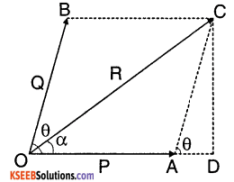P and Q are two forces acting at a point O, making an angle θ. They are represented by OA and OB. the diagonal OC of the parallelogram OBCA represents the resultant R.
Draw CD perpendicular to extended line of OA. In the right angle triangle ODC.
OC² = QD² + CD²
= (OA + AD)² + CD²
= OA² + 20A. AC cosq + AC²
R² = P² + 2PQcos θ + Q²
AC² = AD²+ CD² & $$\frac{A D}{A C}$$ = cos θ
Magnitude of the resultant
R = $$\sqrt{\mathrm{P}^{2}+\mathrm{Q}^{2}+2 \mathrm{PQ} \cdot \cos \theta}$$
To find the direction:
If a is the angle made by R with P, then from the right angled triangle ODC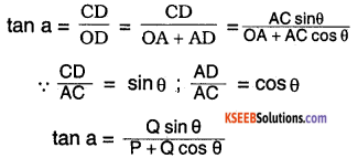Question 10.
A projectile shot at an angle of 60° above the horizontal ground strikes a vertical wall 30 m away at a point 15m above the ground. Find the speed with which the projectile was launched and the speed with which it strikes the wall. Take g = 10 ms-2
Let the initial velocity of the projectile be u
Horizontal component = ux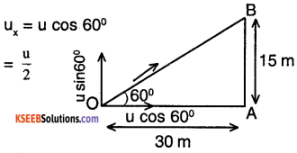Horizontal distance, d = ux t
i.e., 30 = $$\frac{\mathrm{u}}{2}$$ t
⇒ t = $$\frac{60}{\mathrm{u}}$$ ………… (1)
Vertical component uy = usin60°
= $$\frac{\mathrm{u} \sqrt{3}}{2}$$
Vertical displacement s = uyt – 1/2 g t²
15= $$\frac{\mathrm{u} \sqrt{3}}{2}$$ t – 1/2 × 10 × t²
Substitute t = 60/u from (1),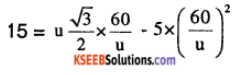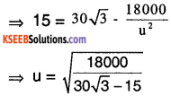⇒ u = 22.07 ms-1
∴Speed of launch = 22.07 ms-1
At the time of striking the wall,
vx = ux = 22.07 cos 60°
= 11.04 ms-1
vy = uy – gt
= 22.07 sin 60° – 10 × $$\left(\frac{60}{22.07}\right)$$
vy = -8.07 ms-1
Resultant speed v = $$\sqrt{v_{x}^{2}+v_{y}^{2}}$$
= $$\sqrt{11.04^{2}+(-8.07)^{2}}$$
= 13.68 ms-1</sup

Question 11.
Define projectile. Show that the path of projectile is a parabola. Find the angle of projection at which the horizontal range and maximum height of the projectile are equal.
Consider a particle thrown up at an angle q to the horizontal with a velocity u. The velocity of projection can be resolved into two component u cos θ and u sin θ along OX and OY respectively.The horizontal component remains constant while the vertical component is affected by gravity.
Let P(x, y) be the position of the projectile after ‘t’ seconds.
Then distance travelled ‘x’ along OX is given by,
x = horizontal component of the velocity × time
x = ucos θ × t …………………. (1)
The distance travelled along the vertical direction in same time ‘t’ is,
y = (usin θ) t – 1/2gt² ………… (2)
Substituting, t = $$\frac{x}{u \cos \theta}$$ from (1) in (2),y = ax – bx² …………………… (3) where
a = tan θ, b = $$\frac{\mathrm{g}}{2 \mathrm{u}^{2} \cos ^{2} \theta}$$, are constants
for given values of θ, u and g.
Equation (3) represents a parabola. Therefore trajectory of a projectile is a parabola.

1st PUC Physics Motion in a Plane Numerical Problems Questions and Answers

Question 1.
A ball is thrown Into air with a speed of 62 ms-1 at an angle of 45° with the horizontal. Calculate

1. the maximum height attained
2. the horizontal range
3. the time of flight and
4. the velocity of the ball after 4 seconds.

Solution:
1. Maximum height, H = $$\frac{u^{2} \sin ^{2} \theta}{2 g}$$ Here,
u = 62 ms-1, θ = 45°, g = 9.8 ms-2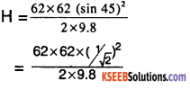= $$\frac{62 \times 62}{4 \times 9.8}$$ = 98.06 m.

2. Horizontal range. R is given by,
R = $$\frac{u^{2} \sin 2 \theta}{g}$$
= $$\frac{62 \times 62 \times \sin 90}{9.8}$$
= $$\frac{62 \times 62}{9.8}$$ = 392.2m.

3. The time of flight,
T = $$\frac{2 u \sin \theta}{g}$$
= $$\frac{2 \times 62 \times \sin 45}{9.8}$$
= $$\frac{2 \times 62}{\sqrt{2} \times 9.8}$$ = 8.95 s.

4. The horizontal component of the velocity remains constant throughout.
∴ Horizontal component of the velocity after 4 seconds vx = ucos θ
Here u = 62 ms-1 and θ = 45°
∴ vx = 62 cos45° = $$\frac{62}{\sqrt{2}}$$ = 44.3 s.
The vertical component of the velocity changes due to the acceleration due to gravity.
We have vy = uy + at,
Here, uy = usin θ; a = -g; t = 4 s;
From vy = usin θ – 4g
∴ vy = 62 sin45 – 9.8 × 4
= $$\frac{62}{\sqrt{2}}$$ -39.2 = 4.64 ms-1
Magnitude of the resultant velocity after 4 seconds is,
v = $$\sqrt{v_{x}^{2}+v_{y}^{2}}$$
= $$\sqrt{(44.3)^{2}+(4.64)^{2}}$$ = 44.5 ms-1
Direction of the velocity is given by,
tan a = $$\frac{v_{y}}{v_{x}}$$ = $$\frac{4.64}{44.3}$$ = 0.104
∴ a = tan-1(0.104) = 5°5’

Question 2.
A bullet is fired at an angle of 60° with the vertical with certain velocity hits the ground 3 km away is it possible to hit the target 5 km away by adjusting the angle of projection? If not, what must be the velocity of projection for the same angle of projections?
Solution:
Let ‘u’ be the velocity of projection.
Angle of projection ‘θ’ = 90 – 60° = 30°
Horizontal Range,
R = $$\frac{u^{2} \sin 2 \theta}{9}$$ = $$\frac{u^{2} \sin 60}{g}$$
∴ u2 = $$\frac{\mathrm{Rg}}{\sin 60}$$ …………… (1)
For a given velocity, the maximum range attained is given by making sin2 θ =1, and
Rmax $$\frac{u^{2}}{g}$$.
Substituting for u² from (1), we have
Rmax = $$\frac{\mathrm{R}}{\sin 60}$$
= $$\frac{3}{0.866}$$ = 3.46km
Since the maximum range is less than 5 km, it is not possible to hit the target 5km away.
From equation (1), we have,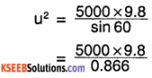= 56582
∴ u = 237.9 ms-1
Thus the bullet will hit the target if it is projected with a velocity of 237.9 ms-1

Question 3.
A stone of mass 0.5 kg is tied to one end of a string and whirled along the horizontal circle of 1.2 m radius, If the period of rotation is 5 s, calculate the tension in the string. If breaking tension is 1.5 N, calculate the maximum speed with which it is rotated and also calculate the corresponding period of rotation.
Solution:
When the mass is rotated along a horizontal circle, the centripetal force is equal to the tension of the string.
Hence, T= mw²r,
Here, m = 0.5 kg,
w = $$\frac{2 \pi}{T}$$ = $$\frac{2 \pi}{5}$$
r = 1.2 m.
∴ T = 0.5 × $$\left(\frac{2 \pi}{5}\right)^{2}$$ × 1.2
= $$\frac{0.5 \times 4 \pi^{2} \times 1.2}{25}$$
= 0.9475 N
The maximum tension which the string can withstand without breaking is 1.5 N and the corresponding velocity ‘v’ is given by, 1.5 = $$\frac{m v^{2}}{r}$$
∴ v2 = $$\frac{1.5 \times r}{m}$$ or
v = $$\sqrt{\frac{1.5 \times 1.2}{0.5}}$$
= 1.89 ms-1
The corresponding angular velocity is given by,
w = $$\frac{\mathrm{v}}{\mathrm{r}}$$ = $$\frac{1.89}{1.2}$$
∴ The period of rotation,
T = $$\frac{2 \pi}{1.575}$$ = 3.99 s.

Question 4.
At what angle should a cyclist lean over from the vertical while negotiating a curve of radius 58m with a speed of 15 km/hour?
Solution:
The angle of banking is given by,
tan θ = $$\frac{v^{2}}{r g}$$
Here, v = $$\frac{15000}{60 \times 60}$$ = 4.17 ms-1
∴ tan θ = $$\frac{(4.17)^{2}}{58 \times 9.8}$$ = 0.03059
θ = tan-1(0.03059)
= 1°45’.Question 5.
A train is moving round a curve of 52m radius and the distance between the rails is 1.2 m by how much should the outer rails be raised above the inner one so that a train running at the rate of 45 kmhr-1 may not skid.
Solution:
The angle of banking is given by,
tan θ = $$\frac{v^{2}}{r g}$$
Here, v = $$\frac{45000}{60 \times 60}$$ = 12.5 ms-1 and
r = 52 m
∴ tan θ = $$\frac{(12.5)^{2}}{52 \times 9.8}$$ = 0.3066
θ = tan-1(0.3066)
= 17°2’
If ‘h’ is the height of the outer rail above the inner one, then
h = 1.2’sin 17.05°
= 0.352 m.

Question 6.
A golf ball leaves the tee with a velocity of 50ms-1 at an angle of 300 with the horizontal. Find its

1. time of flight
2. Range of projectile and
3. The velocity with which it hits the ground at the end of its flight.

u = 50m/s, θ = 30°.
1. Time of flight
T = $$\frac{2 u \sin (\theta)}{g}$$ = $$\frac{2 \times 50 \times \sin (30)}{9.8}$$ = 5.10 s

2. Range R = $$\frac{u^{2} \sin (2 \theta)}{g}$$ = $$\frac{50^{2} \sin \left(2 \times 30^{\circ}\right)}{9.8}$$ = 220.92 m

3. Velocity at the end = velocity of projection = 50 m/s

Question 7.
The greatest and the least resultants of two forces acting at a point are 5 N and1N respectively. Find the forces. What is the resultant of these two forces when they act at an angle of 50°?
Solution:
Let P and Q be the two forces. The greatest resultant is
P + Q = 5 …………… (1)
The minimum resultant is,
P – Q = 1…………….. (2)
2P = 6
P =3N
∴ Q = 2 N
When they are acting at an angle,
θ =50°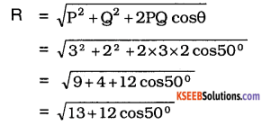= 4.55 N
The direction of the resultant is,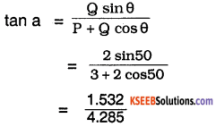= 0.3575
∴ a = 19.67° = 19° 40′
Therefore the resultant is making an angle of 19°40′ with 3N.

Question 8.
Four forces of magnitudes 2N, 3N, 4N, and 5N are acting on a body at a point are inclined at 30°, 60°, 90°, and 120° respectively with the horizontal. Find their resultant.
Solution: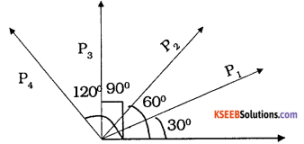Given, P1 =2N, P2 = 3N
P3 = 4N and P4 = 5N
These forces can be resolved into components along the X and Y directions.
Rx= P1 cos θ1 + P2 cos θ2 + P3 cos θ3 + P4 cos θ4
= 2cos 30° + 3cos 60 + 4cos 90 + 5 cos 120
= 2$$\frac{\sqrt{3}}{2}$$ + 3 × $$\frac{1}{2}$$ + 4 × 0 + 5 × $$\frac{1}{2}$$ = 0.732 N

Ry= P1 sin θ1 + P2 sin θ2 + P3 sin θ3 + P4 sin θ4
= 2 sin 30 + 3 sin 60 + 4 sin 90 + 5 cos 120
= 2 × $$\frac{1}{2}$$ + 3 × $$\frac{\sqrt{3}}{2}$$ + 4 × 1 + 5 × $$\frac{\sqrt{3}}{2}$$
= 1 + 1.5$$\sqrt{3}$$ + 4 + 2.5$$\sqrt{3}$$
= 1 + 2.59 + 4 + 4.33
= 11.92 N
∴ Resultant force R = $$\sqrt{\mathrm{R}_{\mathrm{x}}^{2}+\mathrm{R}_{\mathrm{Y}}^{2}}$$
= Vo.7322 +11.92 2
= 11.96 N.

Question 9.
The resultant of two forces acting at 60° is $$\sqrt{13}$$ N. When the same forces act at 90° the resultant is $$\sqrt{10}$$ N. Find the magnitude of the two forces.
Solution:
Let P and Q be the two forces. When the angle between them is 60°, resultant force is $$\sqrt{13}$$ N. Thus
$$\sqrt{\mathrm{P}^{2}+\mathrm{Q}^{2}+2 \mathrm{PQ} \cos 60^{\circ}}$$ = $$\sqrt{13}$$
i.e.$$\sqrt{\mathrm{P}^{2}+\mathrm{Q}^{2}+\mathrm{PQ}}$$ = $$\sqrt{13}$$
P + Q + PQ = 13 ……………(1)
When the angle is 90° resultant force is
$$\sqrt{10}$$ N.
$$\sqrt{\mathrm{P}^{2}+\mathrm{Q}^{2}+2 \mathrm{PQ} \cos 90^{\circ}}$$ = $$\sqrt{10}$$
or $$\sqrt{\mathrm{P}^{2}+\mathrm{Q}^{2}}$$ = $$\sqrt{10}$$
P2+ Q2 =10 ……………….. (2)
Substituting (2) in (1)
10 + P = 13
PQ = 3 ……………………(3)
(P + Q)² = P²+ Q² + 2PQ
= 10 + 2 × 3 = 16
P + Q =4 ………………. (4)
(P – Q)² = P² + Q² – 2PQ
= 10 – 6 = 4
P – Q = 2 ………………. (5)
2P =6; 2Q = 2
P = 3N and Q = 1N.Question 10.
The sum of the two forces is 8 kg wt and the magnitude of the resultant which is at right angles to the smaller force is 4 kgwt. Find the two forces.
Solution:
Let P be the smaller force.
P + Q = 8 kg wt.,
a = 90°, R = 4 kg wt.,
tan α = $$\frac{\mathrm{Q} \sin \theta}{\mathrm{P}+\mathrm{Q} \cos \theta}$$
tan 90° = $$\frac{\mathrm{Q} \sin \theta}{\mathrm{P}+\mathrm{Q} \cos \theta}$$
∞ = $$\frac{\mathrm{Q} \sin \theta}{\mathrm{P}+\mathrm{Q} \cos \theta}$$
⇒ P + Q cos θ = 0
cos θ = $$-\frac{P}{Q}$$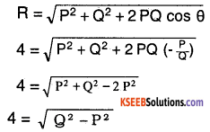Q² – P²= 16
(Q-P)(Q+P) = 16
(Q-P) × 8 = 16
Q – P = 2
P + Q = 8
∴ 2Q = 10 ⇒ Q = 5 kg wt. and P = 3 kg wt.

Question 11.
The resultant of two forces acting at an angle of 150° is perpendicular to the smaller force. If the smaller force is 12$$\sqrt{3}$$ N, find the greater force and its resultant.
Solution:
θ = 150°,
Let P be the smaller force P = 12$$\sqrt{3}$$ N
a = 90°, Q = ?, R = ?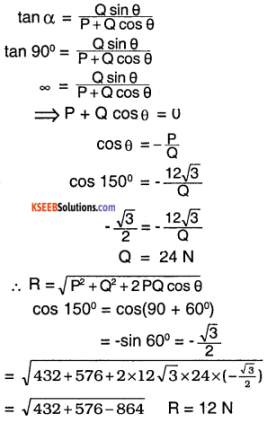Question 12.
Two equal, non parallel forces acting at a point on a body. The square of the resultant is found to be three times the product of the forces, what is the angle between them.
Given, P = Q & R² = 3PQ = 3P²
we have R² = P² + Q² + 2PQ cos θ
i.e. 3P²= P² + P² + 2P² cos θ
i.e. 3P² = 2P² + 2P² cos θ
1P² = 2P²cos θ
$$\frac{1}{2}$$ = cose
⇒ θ = cos-1($$\frac{1}{2}$$) = 60°.

Question 13.
The greatest and least resultant of two forces acting at a point are 17N and 7N respectively. Find the two forces. If these forces act at right angles, find the magnitude of their resultant.
For maximum resultant
P + Q=17N ……………….. (1)
For minimum resultant
P – Q = 7N ……………….. (2)
Eq(1) + (2) gives 2P = 24 Or P = 24/2 = 12N.
From (1), Q = 17 – 12 = 5N
When P and Q are in perpendicular directions,
R = $$\sqrt{\mathrm{P}^{2}+\mathrm{Q}^{2}}$$
= $$\sqrt{12^{5}+5^{2}}$$ = 13N(∵ cos 90° = 0).

Question 14.
Two equal forces are acting at a point and angle between them in 60°. Calculate magnitude of Resultant of these forces.
Q = P and θ = 60°.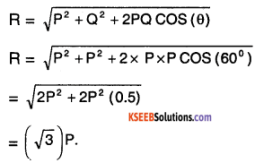1st PUC Physics Motion in a Plane Hard Questions and Answers

Question 1.
The total speed v1 of a projectile at its greatest height is $$\sqrt{\frac{6}{7}}$$ of its speed v2 at half its greatest height. Find the angle of projection.
Velocity at the highest point = ucos θ = v1
Hmax = $$\frac{\mathrm{u}^{2} \sin ^{2} \theta}{2 \mathrm{g}}$$
Using v = u – 2gh,
At h = $$\frac{\mathrm{H}_{\mathrm{max}}}{2}$$, vertical component of v2 is
given by,
v2y2 = u2sin2θ – 2g × $$\frac{\mathrm{H}_{\mathrm{max}}}{2}$$
v2y2 = u2 sin2θ – $$\mathrm{g}\left(\frac{\mathrm{u}^{2} \sin 2 \theta}{2 \mathrm{g}}\right)$$
⇒ v = $$\frac{\mathrm{u}^{2} \sin ^{2} \theta}{2}$$
Horizontal component v2 is
v2x = v1 = ucosθ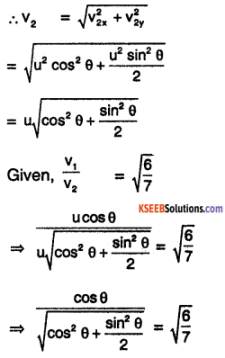Squaring both sides,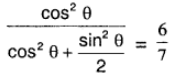⇒ 7 cos²θ = 6 cos²θ + 3 sin² θ
⇒ cos²θ = 3 sin²θ
⇒ tan²θ = 1/3
⇒ tan θ = ± $$1 / \sqrt{3}$$
⇒ θ = ± 30°
θ = 30°
(because negative angle is not possible)

Question 2.
Prove that the path of one projectile as seen from another projectile is a straight line.
The coordinates of first projectile as seen from the second projectile are
X = x1 – x1
= u1cos θ1 t – u2cos θ2 t
X = (u1cos θ1 – u2cos θ2)t
Y = y1 – y2
= u1sin θ1 t – 1/2 gt² – (u2sin θ2 t – 1/2 gt²)
Y = (u1 sin θ1 – u2sin θ2)t
∴ $$\frac{Y}{X}=\frac{\left(u_{1} \sin \theta_{1}-u_{2} \sin \theta_{2}\right) t}{\left(u_{1} \cos \theta_{1}-u_{2} \cos \theta_{2}\right) t}$$
= a constant, say m
This equation is of the form Y = mX, which is the equation of a straight line. Thus the path of a projectile as seen from another projectile is a straight line.

Question 3.
A particle is projected with some speed at an angle a to the horizontal from the end B of the horizontal base BC of a triangle ABC. It rises to the vertex A and after just grazing it, falls down to reach point C of the base BC. If the base angles of the triangle are β and γ, show that 4 cot α = cot β + cot γ.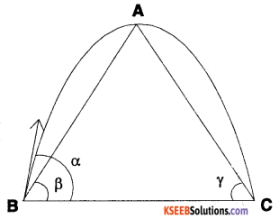cot β = $$\frac{\mathrm{BD}}{\mathrm{h}_{\max }}$$
and cot γ = $$\frac{\mathrm{DC}}{\mathrm{h}_{\max }}$$
cot β + cot γ = $$\frac{\mathrm{BD}+\mathrm{DC}}{\mathrm{h}_{\max }}$$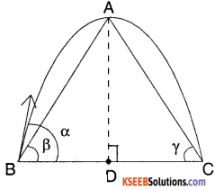$$\frac{\text { Range }}{h_{\max }}$$
⇒ cot β + cot γ = $$\frac{\left(u^{2} \sin 2 \alpha\right) / g}{\left(u^{2} \sin ^{2} \alpha\right) / 2 g}$$
⇒ cot β + cot γ = 2 × $$\frac{2 \sin \alpha \cos \alpha}{\sin ^{2} \alpha}$$